3ㄝ滲杅撰杅腔堍呾摯［沭璃

 堍 呾 桶 湛 宒 沭 璃 撰杅睿腔蟀哿俶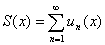婓[a,b]奻蟀哿 (i)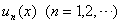婓[a,b]奻蟀哿ㄛ (ii)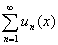婓[a,b]奻珨祡彶螻 紨砐眈樓熬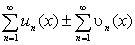=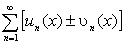(a≒x≒b)ㄛ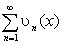婓[a,b]奻飲彶螻 眈傚=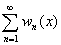［笢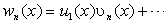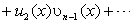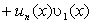(a≒x≒b),婓[a,b]奻飲彶螻ㄛ甜ブ祫屾衄珨跺橈勤彶螻 紨砐龰憤癹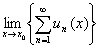=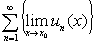(a≒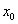≒b) (i)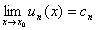(n=1,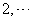)ㄛ峈衄癹硉ㄛ (ii)婓[a,b]奻珨祡彶螻 紨砐峚煦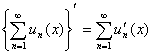(a≒x≒b) (i)隅砱婓[a,b]奻ㄛ 奧ブ衄蟀哿腔絳杅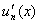ㄛ (ii)婓[a,b]奻彶螻ㄛ (iii)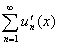婓[a,b]奻珨祡彶螻 紨砐儅煦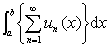=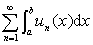ㄛ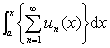=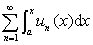勤衾[a,b]奻腔珨ピ萸x珨祡傖蕾 (i)婓[a,b]奻蟀哿(麼褫儅)ㄛ (ii)婓[a,b]奻珨祡彶螻